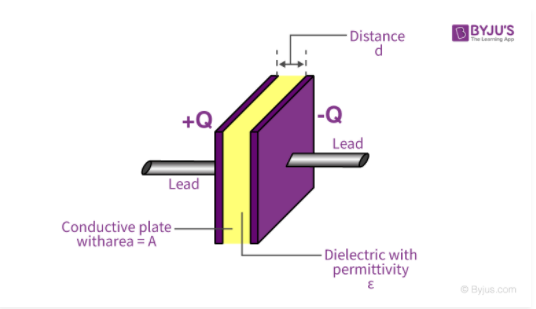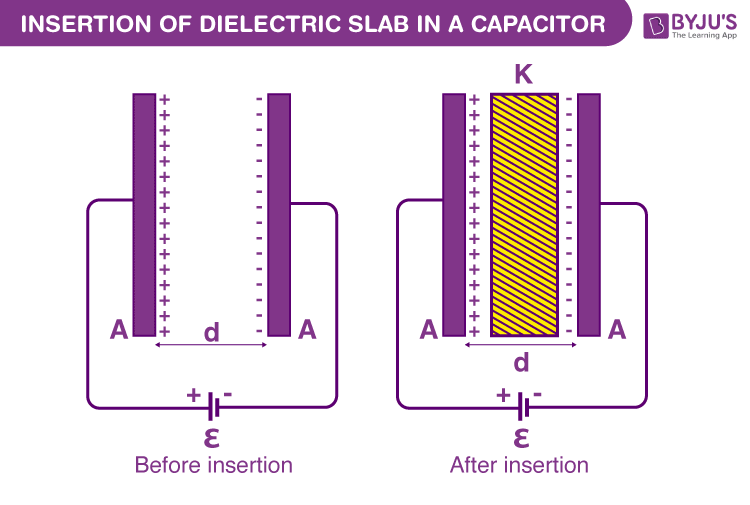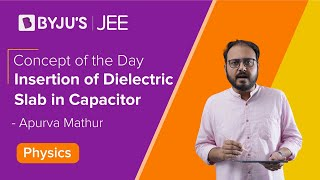# Insertion of Dielectric Slab in Capacitor

The insertion of a dielectric slab in a capacitor will polarise the charges. The polarization of the charges on either side of the dielectric will produce an electric field in a direction opposite to the field produced by the source. The net electric flux will become zero, this effect will result in an increase in capacitance.

## Dielectric

Dielectric are non-conducting materials. It will not have any free charge carriers. In a dielectric, an external electric field will induce a dipole moment by re-orienting the molecules in the dielectric. The collective effect of the molecular dipole moment is that a field is produced that will oppose the external electric field. The opposing field that is produced will not cancel the external field, it will only reduce it. The extent of the effect depends on the type of dielectric used. The dipole moment per unit volume is called polarisation. Examples of dielectrics are plastic, glass, porcelain, mica and metal oxides.

## Dielectric Constant

The dielectric constant is given by the ratio of the permittivity of the dielectric material to the permittivity of the vacuum. Permittivity is the ability of a dielectric to store electric energy in the electric field by the polarization of the material. Permittivity is generally expressed as the relative quantity, it is the ratio of the permittivity of the material to the vacuum permittivity. Since the dielectric constant is the ratio of two similar quantities, it will not have any unit or dimension. The dielectric constant is expressed as k.

Dielectric constant, k = ε/ε0

ε is the permittivity of the dielectric

ε0 is the permittivity of vacuum

## Capacitor and CapacitanceA capacitor is a system of two parallel plate conductors. In practice, the two parallel conductors will have a charge of -Q and +Q. The potential difference between the two capacitors is given as V = V1 – V2, here V1 and V2 are the potential of the conducting plate 1 and conducting plate 2, respectively. The capacitor is charged by connecting the two conducting plates to the two terminals of the battery. The charge of the capacitor is taken as Q, though it is the charge on one of the conductors and the total charge of the capacitor will be zero.

The electric field between the plates of the conductor is proportional to the charge Q. This means if the charge on the capacitor is increased, the electric field will also be increased at all points. The potential difference between the two plates can be defined as the work done per positive charge in taking a small test charge from one conductor to the other against the electric field. Therefore, the potential difference V is proportional to the charge Q. The ratio of Q/V is proportional to a constant C.

Capacitance, C = Q/V

Here, Q is the charge of the capacitor

V is the potential difference between the plates of the capacitor

The constant C is called the capacitance of the capacitor. The capacitance depends on the size, shape and separation between the plates. If the capacitor has a large capacitance, it means that the capacitor can hold a large amount of charge at a relatively smaller potential difference.

## Parallel Plate Capacitor

Let us consider the intervening medium between the two conducting plates of the capacitor to be the vacuum. The area of the plates is taken as A and d is the distance of separation between the plates. The surface charge density of the plates is σ = Q/A. The total electric field between the two plates will add up, giving

E = (σ/2ε0) + (σ/2ε0) = σ/ε0

= (Q/Aε0)

The potential difference between the plates is equal to the electric field times the distance between the plates.

V = Ed = (Q/Aε0) d

The capacitance C of the parallel plate capacitor can be written as

C = Q/V = Aε0/d

The energy U stored in the capacitor is the electrostatic potential energy and it is related to the capacitance and the voltage.

U = (½) CV2

## Insertion of Dielectric slab in a capacitorWhen a dielectric slab is inserted between the plates of the capacitor connected to a battery, the dielectric will get polarised by the field. This will produce an electric field inside the capacitor, directed opposite to the direction of the external electric field due to the battery. The result is that the net effect of the electric field is reduced. This, in turn, will increase the capacitance by a factor of k. The capacitance with a dielectric slab in between is given by

Capacitance, C’ = kQ/V = kAε0/d = kC

Here, k is the dielectric constant

The potential difference V’ and the electric field E’ will remain the same before and after insertion of the dielectric slab between the plates of the capacitor that is connected to a battery.

V’ = V

E’ = E

The charge on the capacitor increases by a factor k after the dielectric is introduced between the plates. The charge after the insertion of the dielectric slab is given as

Q’ = C’V’

= (kC)V (since C’ = kC and V’ = V)

Q’ = kQ

The energy stored when a dielectric slab is inserted is given by

U’ = (½)C’V’2

= (½) (kC)V2 (since C’ = kC and V’ = V)

= k (½)CV2

= kU

The table below shows the change in the different parameters when a dielectric is inserted between the plates of the capacitor connected to the battery.

 Capacitance C = ε0A/d Potential difference V = Q/C Electric Field E = V/d Charge Q = CV Energy U = (½) CV2 Before insertion of dielectric C V E Q U After insertion of dielectric kC V E kQ kU

### Solved Problems

1.A capacitor with capacitance 90pF is connected to a battery of emf 20V. A dielectric material of dielectric constant K = 5/3 is inserted between the plates, the magnitude of the induced charge will be

(a) 0.3 nC

(b) 2.4 nC

(c) 0.9 nC

(d) 1.2 nC

Solution:

Charge of the capacitor without dielectric, Q = CV = 90 x 20 = 1800 pC

Q’ = kCV = (5/3) x 90 x 20 = 3000 pc

Qind = Q’ – Q = 3000 pC – 1800 pC = 1200 pC = 1.2 nC

Related Video